# Tuesday Homework (due 9am on Thursday) Combinatorial Optimization

 Tuesday Homework (due 9am on Thursday) Combinatorial Optimization (Clifford Stein) Problem 1 (10 points) Prove that given any flow network G, there exists a sequence of at most E augmenting paths that will lead to the maximum flow. (Hint: no known algorithm can find a flow using just E augmenting paths. Think about finding the set of paths after you have already computed a maximum flow via some other means.) Problem 2 (10 points) Professor May B. Wright claims that the residual graph is unnecessarily complicated and proposes that you can find a maximum flow by finding a series of augmenting paths in a modified residual graph which does not include any of the reverse edges.  Show that the Professor is incorrect by giving a set of augmenting paths in the modified residual graph that does not lead to the maximum flow.  That is, you should have a flow such that there are no augmenting paths in the modified residual graph, yet the flow is not maximum. (Extra credit:  How large can you make the gap between this type of flow and a real maximum flow). TSP and Bin Packing (David Johnson) Problem 1 (10 points) Prove that the asymptotic worst-case ratio for the Next Fit bin packing algorithm is 2. That is, prove that for all lists L of items, NF(L) ≤ 2OPT(L), and show examples of lists Ln such that limit (NF(Ln)/OPT(Ln)) = 2. Problem 2 (10 points) Prove that if an undirected graph is connected and every vertex has even degree, it contains an Euler tour that can be found in linear time, where an "Euler tour" is a path through the graph that traverses each edge exactly once. Shortest Paths (Renato Werneck) (10 points) Let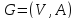be a directed graph with positive edge lengths. For vertices a and b, let dist(a, b) be the length of a shortest path from a to b in G. Assume all shortest paths in G are unique. Given two vertices s and t, let Pst be the shortest path between s and t. For any vertex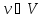, its reach (denoted by r(v)) is the maximum, over all shortest paths Pst that contain v, of min{dist(s, v), dist(v, t)}. We are interested in solving the verification problem. If someone claims to have computed valid nonnegative upper bounds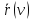for all reaches, we would like to check if indeed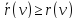for every vertex. As stated, this problem can be easily solved by growing (with Dijkstra’s algorithm) a shortest path tree Ts from each vertex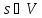. For each tree Ts, compute for each node v its depth ds(v) (the distance from the root s to v) and its height hs(v) (the distance from v to its farthest descendant). If the reach upper bounds are correct, we must always have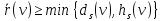. Give a polynomial algorithm to solve the verification problem if the original shortest paths in G are not necessarily unique (i.e., when there may be multiple shortest paths between any two vertices). More precisely, given a graphand nonnegative boundsfor all vertices v, your algorithm must decide whether the following statement is true: For every pair (s, t), there exists at least one shortest path Pst such that, for every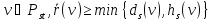. The following hints may be useful: 1. The algorithm above (running Dijkstra n times) still works, as long as it uses the appropriate tie-breaking rule to choose among candidate parent vertices. Find this rule. 2. To implement such a rule, you may want to maintain an auxiliary value with each vertex.Download 34.88 Kb.Share with your friends: